# SSC Reasoning Ability Practice Questions – (Day-1)

Dear Aspirants, Here we have given the Important SSC CGL Exams 2018 Practice Test Papers. Candidates, those who are preparing for SSC CGL Exams 2018 can practice these questions to get more confidence to Crack SSC CGL Exams 2018 Examination.

[WpProQuiz 3984]

1) Sumitra remembers that her mother’s birthday is after 13th February but before 16th February but her brother remembers that his mother’s birthday is after 14th February but before 17th February. On which date Sumitra’s mother’s birthday will be celebrated?

a) 13 February

b) 14 February

c) 15 February

d) 17 February

2) There are five energy drinks – Red, Moto, Energy, Lion and Bull containing the different range of sugar content. Moto having sugar content more than all other drinks. Energy having the sugar content only more than the Lion. Bull is not having sugar content more than the Red. Which of the following drink having the second most sugar content?

a) Bull

b) Energy

c) Red

d) Moto

Directions (3 – 4): In the following questions, select the odd word/ letter/ number pair from the given alternatives.

4.

a) AZB

b) LOQ

c) EVX

5.

a) 41 – 22

b) 63 –93

c) 82 – 44

d) 83 – 64

5) In the question, two statements are given, followed by two Conclusions, I and II. You have to consider the statements to be true even if it seems to be at variance from commonly known facts. You have to decide which of the given conclusions, if any, follows from the given statements

Statements:

All steel is iron.

No iron is solid.

Conclusions:

I. No steel is solid.

II. Some iron is steel

a) Only Conclusion I follows.

b) Only Conclusion II follows.

c) Both Conclusion I and II follow.

d) Neither Conclusion I nor II follows.

6) If a mirror is placed on the line MN, then which of the answer figures is the right image of the given figure?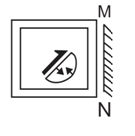a)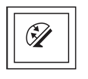b)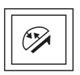c)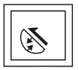d)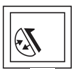7) A word is represented by only one set of numbers are given in any one of the alternatives. The sets of numbers given in the alternatives are represented by two classes of alphabets as shown in the given two matrices. The columns and rows of Matrix – I are numbered from 0 to 4 and that of Matrix-II are numbered from 5 to 9. A letter from these matrices can be represented first by its row and next by its column, for example, ‘Y’ can be represented by 55, 87 etc., and ‘Z’ can be represented by 14, 01 etc. Similarly, you have to identify the set for the word ‘VERY’.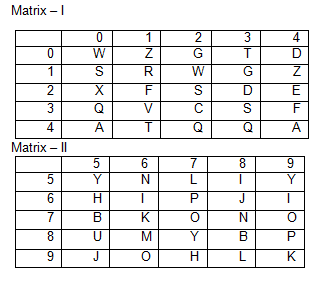a) 01, 43, 21, 78

b) 31, 24, 11, 87

c) 11, 20, 66, 85

d) 88, 56, 43, 20

8) Arrange the given words in the sequence in which they occur in the dictionary.

1) Dillydallying

2) Dillydallied

3) Dillydally

4) Dilled

a) 4, 2, 3, 1

b) 4, 2, 3, 1

c) 4, 3, 1, 2

d) 4, 3, 2, 1

9) In the following question which one set of letters when sequentially placed at the gaps in the given letter series shall complete it?

lm_o_nm_l_n_ _nml

a) molnon

b) nolmoo

c) nomloo

d) noolmm

10) If 6 * 9 – 4 = 58 and 3 * 9 – 7 = 34, then in the expression A * 4 –91, what is the value of ‘A’?

a) 6.5

b) 17.5

c) 20.5

d) 30.5

11) Two positions of a cube are shown below. What will come opposite to face containing ‘4’?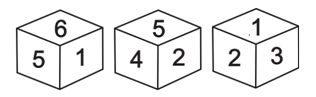a) 1

b) 2

c) 3

d) 4

12) Identify the diagram that best represents the relationship among the given classes.

Green, Mango, Fruits

a)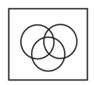b)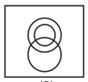c)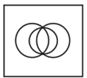d)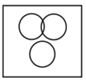13) Which answer figure will complete the pattern in the question figure?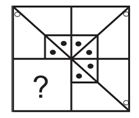a)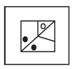b)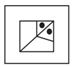c)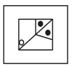d)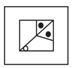14) If a mirror is placed on the line AB, then which of the answer figure is the right image of the given figure?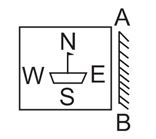a)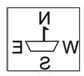b)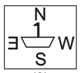c)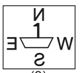d)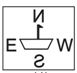15) If ‘’ denotes ‘subtracted from’, ‘?’ denotes ‘multiplied by’, ‘?’ denotes “added to” and ‘?’ denotes “divided by”, then the value of 10?8?4?89

a) 7

b) 5

c) 9

d) 11

According to Sumitra      14 / 15

According to her brother  15 / 16

From both conditions commo,n date is 15.

Hence, the birthday of Sumitra’s mother is on 15 February.

Moto > Red > Bull > Energy > Lion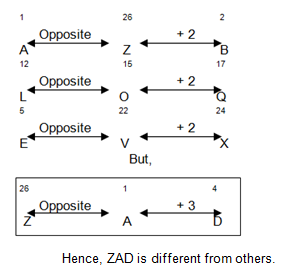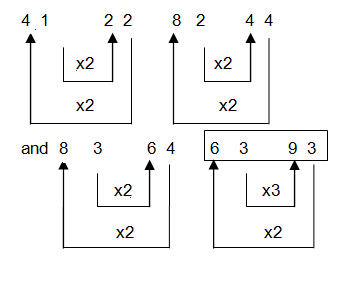According to statements,

Venn diagram is as follow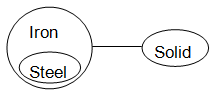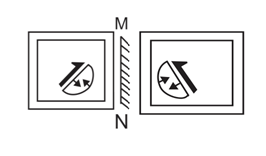According to the question

Hence, VERY = 31, 24, 11, 87

4. Dilled

2. Dillydallied

3. Dillydallied

1.Dillydallying

Order of series is as follows

l m n o o n m l / l m n o o n m l

On placing set ‘nolmoo’ into the gaps sequentially it will complete the series.

As, (6*9) + \$ = 54 + 4 = 58

And (3*9) + 7 = 27 + 7 = 34

Similarly, (A * 4) + 9 = 91

4A = 91 – 9 = 82

A = 20.5

From Cube I and II,

Cube Figure I. 5⇒6⇒ 1

Cube Figure II.5⇒2⇒ 4

Opposite

Hence number I will be opposite to the number 4.

According to statement venn diagram is as follows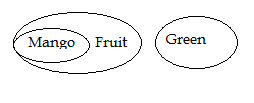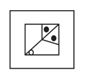On placing the mirror on line AB question figure will appear as answer figure (3).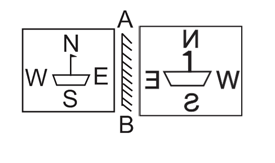Given expression

10?8?4?89

On changing signs

10 + 8 x 4  8 – 9 = ?

According to the BODMAS

10 + 8 X  – 9 = ?

10 + 4 – 9 = ?

14 – 9 = ?

? = 5